# Reasoning Ability Quiz: 4 March 2021

Updated Fri, 05 Mar 2021 02:20 PM IST

Reasoning Ability Quiz For RBI Office Attendant  2021- 4th March

Q:1-5)
Direction: - Study the following information carefully and answer the given question.

Seven people A, B, C, D, E, F, and G are sitting at a circular table. In which some of them are facing towards the center and some of them are facing outward.
B is sitting second to the left of one who is sitting immediate right of C. Only one person sits between F and A, who faces the same direction as A. D is the one who faces outward and setting forth to the right of A. Immediate neighbors of F facing inside. Only one person sits between E and B, who faces towards the center. A is the immediate right of C and C is facing outward.

Q-1) How many persons are facing outward direction?
A) 3
B) 4
C) 5
D) 6
E) None of these

Q-2) who sits second to the right of G?
A) A
B) C
C) F
D) E
E) None of these

Q-3) Four of the following five are alike in a certain way and so form a group.Source: Safalta.com

Which is the one that does not belong to the group?
A) A
B) C
C) G
D) E
E) B

Q-4) Which of the following sits immediately to D?
A) C, A
B) F, E
C) B, E
D) G, F

Q-5) which of the following statement is true?
A) A Sits second right F
B) G is the immediate neighbor of C
C) D sits second to the right of G
D) F and A are facing in the same direction
E) None of the statement is True

Q: 6-8)
Direction: - In the following question below some statements are given followed by some conclusions. Taking the given statements to be true even if they seem to be at variance from commonly known facts, read the entire conclusion and then decide which of the given conclusion logically follows in the given statement.

Q-6)
Statements:
I)Only few Pens are Tab
II)Only few Tab are Word

Conclusion:
I) All Tab can be pen
ll) All Tab can be word

A) Only conclusion, I follows
B)Only conclusion II follows
C)Either conclusion, I or II follows
D)Neither Conclusion I nor II follows
E)Both Conclusion  I and II follows

Q-7)
Statement :
I)Some Red are Blue
II)Only few blue are green

Conclusion:
I)Some Red are not Green
II) All Blue are green is a possibility

A) Only conclusion, I follows
B)Only conclusion II follows
C)Either conclusion, I or II follows
D)Neither Conclusion I nor II follows
E)Both Conclusion  I and II follows

Q-8)
Statement:
All bike are cycle
Only a few cycle are gear

Conclusion:
I)No Bike is gear
II)All the gear can be cycle

A) Only conclusion, I follows
B)Only conclusion II follows
C)Either conclusion, I or II follows
D)Neither Conclusion I nor II follows
E)Both Conclusion  I and II follows

Q: 9-10)
Direction: In the following question assuming the given statement to be true, find which of the following conclusions is/are definitely true and then give your answers

Q-9)
statement : L ≥ M > N = O ; P>O ;  P Conclusion :I) O > T
II) P > N
A) Only conclusion, I follows
B)Only conclusion II follows
C)Either conclusion, I or II follows
D)Neither Conclusion I nor II follows
E)Both Conclusion  I and II follows

Q-10)
Statement : A < B = C ; D ≤ E > F ; D > L ; C > L
Conclusion :   I)E>L
II)L>A

Solutions:

Q: 1-5)

Final Arrangement –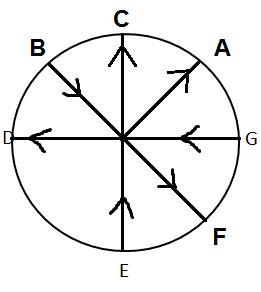Complete solution :
Case-1                                                              Case-2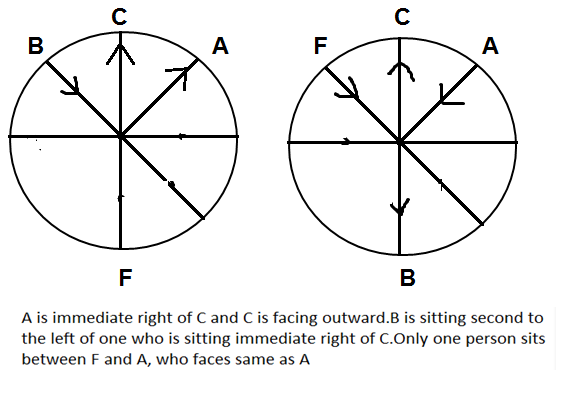Case -1                                                              Case- 2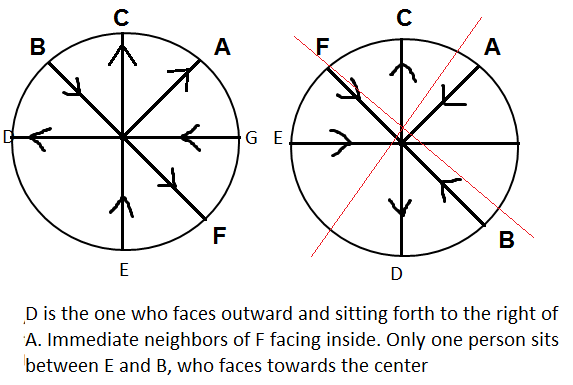Q-1)  B) 4
Q-2)   B) C
Q-3)   C) G
Q-4)  C) B, E
Q-5)  E) None of the statement is True

Q:6)
Ans - A) Only conclusion I follows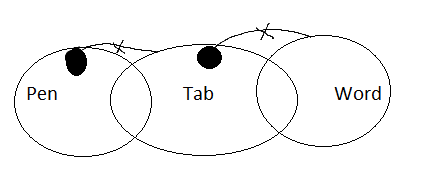I) All Tab can be pen – Follows: as it is possible that all tab can be pen  there is no boundation for tab

II) All Tab can be word – does not follow: as Only few Tab are Word it can’t be possible

Q-7)
ANS - D) Neither Conclusion I nor II follows

I) Some Red are not Green – cant say it can be possible or it cant be possible
II) All Blue are green is a possibility – not follow :  Only few blue are green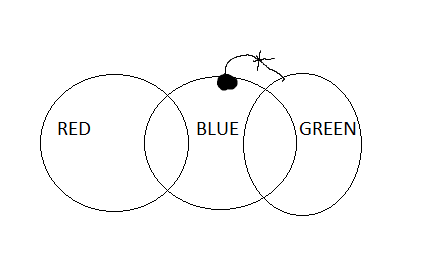Q-8)
ANS - B) Only conclusion II follows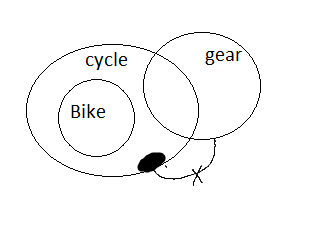Conclusion:

I) No Bike is gear – can’t say : no definite information
II) All the gear can be cycle – Follow : as it is possible

Q-9)
Ans - Only conclusion II follows
Solution
Given statement ; L ≥ M > N = O ; P>O ;  P On combining :  L ≥ M > N = O < P < T
Conclusion :
I) O > T – FALSE  (O < P < T)
II) P > N – TRUE (N = O < P)

Q-10)
ANS – Only conclusion I follows
SOLUTION:
Statement : A < B = C ; D ≤ E > F ; D > L ; C > L
Combining :  A < B = C > L < D ≤ E > F
Conclusion :
I) E>L – TRUE : (L < D ≤ E)
II) L>A – FALSE : (A < B = C > L)